# 通道突破形态

12 二月 2018, 10:05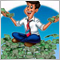1
13 694

### 1. 策略的理论方面

1. 等距通道
2. 标准偏差通道
3. 回归通道
4. 安德鲁斯草叉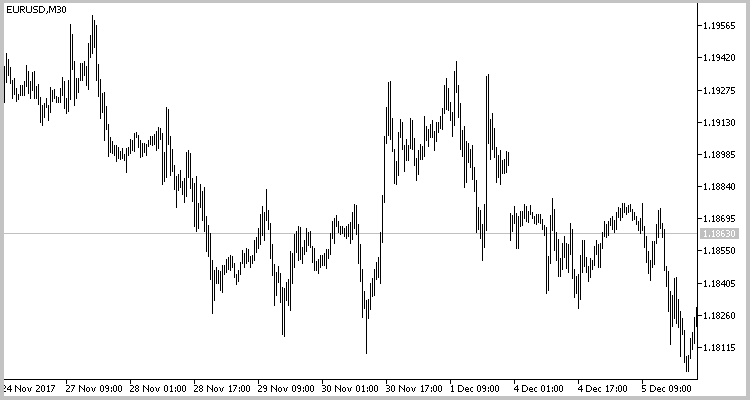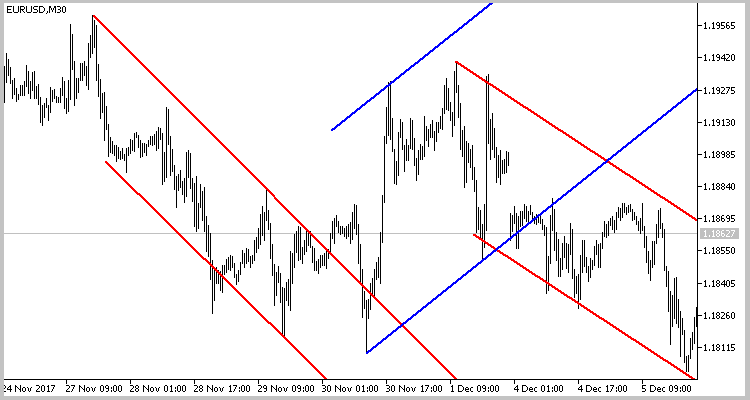### 2. 自动搜索形态

```class CChannel : public CObject
{
private:
string            s_Symbol;      // 品名
ENUM_TIMEFRAMES   e_Timeframe;   // 时间帧
int               i_Handle;      // 指标句柄
datetime          dt_LastCalc;   // 最后计算的柱线
SPeackTrough      PeackTrough[]; // 之字折线峰值数组
int               CurCount;      // 峰值计数
int               PreDir;        // 前一个之字折线的腿方向
int               CurDir;        // 当前之字折线的腿方向
int               RequiredCount; // 通道内的最小峰值数量
double            d_Diff;
bool              b_FoundChannel;
bool              b_Breaked;
datetime          dt_Breaked;
double            d_BreakedPrice;

void              RefreshLast(datetime time,double v);
bool              CheckForm(double base);
double            GetRessistPrice(SPeackTrough &start_peack, datetime time);
double            GetSupportPrice(SPeackTrough &start_peack, datetime time);
bool              DrawChannel(MqlRates &break_bar);
bool              DrawChannel(void);
bool              UnDrawChannel(void);

public:
CChannel(int handle,datetime start_time,string symbol,ENUM_TIMEFRAMES timeframe);
~CChannel();
bool              Calculate(ENUM_ORDER_TYPE &type,double &stop_loss,datetime &deal_time,bool &breaked,datetime &breaked_time);
};

```

```CChannel::CChannel(int handle,datetime start_time,string symbol,ENUM_TIMEFRAMES timeframe) : RequiredCount(4),
CurCount(0),
CurDir(0),
PreDir(0),
d_Diff(0.1),
b_Breaked(false),
dt_Breaked(0),
b_FoundChannel(false)
{
i_Handle=handle;
dt_LastCalc=fmax(start_time-1,0);
s_Symbol=symbol;
e_Timeframe=timeframe;
}

```

UnDrawChannel 函数在类的 deinitialization 函数中调用。它从图表中删除先前添加的图形对象。

```bool CChannel::Calculate(ENUM_ORDER_TYPE &type,double &stop_loss,datetime &deal_time, bool &breaked,datetime &breaked_time)
{
MqlRates rates[];
CurCount=ArraySize(PeackTrough);
if(CurCount>0)
{
dt_LastCalc=PeackTrough[CurCount-1].Bar;
CurDir=PeackTrough[CurCount-1].Dir;
}
int total=CopyRates(s_Symbol,e_Timeframe,fmax(dt_LastCalc-PeriodSeconds(e_Timeframe),0),TimeCurrent(),rates);
if(total<=0)
return false;

```

之后我们初始化返回变量。

```   stop_loss=-1;
breaked=b_Breaked;
breaked_time=dt_Breaked;
deal_time=0;

```

```   for(int i=0;i<total;i++)
{
if(rates[i].time>dt_LastCalc)
{
dt_LastCalc=rates[i].time;
PreDir=CurDir;
}
else
continue;

// 新的最大值

double lhb;
if(CopyBuffer(i_Handle,4,total-i-1,2,lhb)<=0)
return false;

if(lhb!=lhb)
{
if(CurDir==1)
RefreshLast(rates[i].time,rates[i].high);
else
}

// 新的最小值

double llb;
if(CopyBuffer(i_Handle,5,total-i-1,2,llb)<=0)
return false;

if(llb!=llb)
{
if(CurDir==-1)
RefreshLast(rates[i].time,rates[i].low);
else
}

```

```      double base=(CurCount>=2 ? MathAbs(PeackTrough.Val-PeackTrough.Val) : 0);

if(CurCount>=RequiredCount && !b_FoundChannel)
{
if(CurDir!=PreDir)
{
if(CheckForm(base))
{
b_FoundChannel=true;
}
else
{
UnDrawChannel();
dt_LastCalc=PeackTrough.Bar+PeriodSeconds(e_Timeframe);
ArrayFree(PeackTrough);
CurCount=0;
CurDir=0;
PreDir=0;
b_Breaked=false;
dt_Breaked=0;
b_FoundChannel=false;
deal_time=0;
total=CopyRates(s_Symbol,e_Timeframe,fmax(dt_LastCalc,0),TimeCurrent(),rates);
i=-1;
continue;
}
}
}

```

```      if(b_FoundChannel)
{
if(PeackTrough.Dir==1)
{
if(PeackTrough.Val>PeackTrough.Val)
{
if(!b_Breaked)
{
if((rates[i].close-GetRessistPrice(PeackTrough,rates[i].time))>=(d_Diff*base))
{
b_Breaked=breaked=true;
dt_Breaked=breaked_time=rates[i].time;
d_BreakedPrice=rates[i].high;
DrawChannel(rates[i]);
continue;
}
if(CurCount>4 && PeackTrough[CurCount-1].Dir==1 && (GetRessistPrice(PeackTrough,rates[i].time)-PeackTrough[CurCount-1].Val)>0)
{
int channels=ArraySize(ar_Channels);
if(ar_Channels[channels-1]==GetPointer(this))
{
SearchNewChannel(PeackTrough[CurCount-3].Bar-PeriodSeconds(e_Timeframe));
}
}
}
else
{
if(rates[i].time<=dt_Breaked)
continue;
//---
double res_price=GetRessistPrice(PeackTrough,rates[i].time);
if(((rates[i].low-res_price)<=0 && (rates[i].close-res_price)>0 && (rates[i].close-res_price)<=(d_Diff*base)) || rates[i].close>d_BreakedPrice)
{
stop_loss=res_price-base*(1+d_Diff);
deal_time=rates[i].time;
return true;
}
}
}
else
{
UnDrawChannel();
dt_LastCalc=PeackTrough.Bar+PeriodSeconds(e_Timeframe);
ArrayFree(PeackTrough);
CurCount=0;
CurDir=0;
PreDir=0;
b_Breaked=false;
dt_Breaked=0;
b_FoundChannel=false;
deal_time=0;
total=CopyRates(s_Symbol,e_Timeframe,fmax(dt_LastCalc,0),TimeCurrent(),rates);
i=-1;
continue;
}
}
else
{
if(PeackTrough.Val<PeackTrough.Val)
{
if(!b_Breaked)
{
if((GetSupportPrice(PeackTrough,rates[i].time)-rates[i].close)>=(d_Diff*base))
{
b_Breaked=breaked=true;
dt_Breaked=breaked_time=rates[i].time;
d_BreakedPrice=rates[i].low;
DrawChannel(rates[i]);
continue;
}
if(CurCount>4 && PeackTrough[CurCount-1].Dir==-1 && (PeackTrough[CurCount-1].Val-GetSupportPrice(PeackTrough,rates[i].time))>0)
{
int channels=ArraySize(ar_Channels);
if(ar_Channels[channels-1]==GetPointer(this))
{
SearchNewChannel(PeackTrough[CurCount-3].Bar-PeriodSeconds(e_Timeframe));
}
}
}
else
{
if(rates[i].time<=dt_Breaked)
continue;
double sup_price=GetSupportPrice(PeackTrough,rates[i].time);
if(((sup_price-rates[i].high)<=0 && (sup_price-rates[i].close)>0 && (sup_price-rates[i].close)<=(d_Diff*base)) || rates[i].close<d_BreakedPrice)
{
type=ORDER_TYPE_SELL;
stop_loss=sup_price+base*(1+d_Diff);
deal_time=rates[i].time;
return true;
}
}
}
else
{
UnDrawChannel();
dt_LastCalc=PeackTrough.Bar+PeriodSeconds(e_Timeframe);
ArrayFree(PeackTrough);
CurCount=0;
CurDir=0;
PreDir=0;
b_Breaked=false;
dt_Breaked=0;
b_FoundChannel=false;
deal_time=0;
total=CopyRates(s_Symbol,e_Timeframe,fmax(dt_LastCalc,0),TimeCurrent(),rates);
i=-1;
continue;
}
}
}
}
return b_Breaked;
}

```

CChannel 类的完整代码及其函数如下所示。

### 3. 为策略测试创建智能交易系统

```#resource "\\Indicators\\ZigZags\\iUniZigZagSW.ex5"
#include <\\Break_of_channel_DNG\\Channel.mqh>

```

```input ESorce               SrcSelect      =  Src_HighLow;
input EDirection           DirSelect      =  Dir_NBars;
input int                  RSIPeriod      =  14;
input ENUM_APPLIED_PRICE   RSIPrice       =  PRICE_CLOSE;
input int                  MAPeriod       =  14;
input int                  MAShift        =  0;
input ENUM_MA_METHOD       MAMethod       =  MODE_SMA;
input ENUM_APPLIED_PRICE   MAPrice        =  PRICE_CLOSE;
input int                  CCIPeriod      =  14;
input ENUM_APPLIED_PRICE   CCIPrice       =  PRICE_TYPICAL;
input int                  ZZPeriod       =  50;

```

EA 有四个全局变量。在这些变量中写入以下内容:

• 指标句柄,
• 指向通道的指针数组 (CChannel 类的对象),
• 柱线的开盘时间, 最后一次突破发生的时间。
```int         zz_handle;
CChannel   *ar_Channels[];
datetime    dt_last_break;

```

```int OnInit()
{
//---
zz_handle=iCustom(Symbol(),Period(),"::Indicators\\ZigZags\\iUniZigZagSW",SrcSelect,
DirSelect,
RSIPeriod,
RSIPrice,
MAPeriod,
MAShift,
MAMethod,
MAPrice,
CCIPeriod,
CCIPrice,
ZZPeriod);

if(zz_handle==INVALID_HANDLE){
return(INIT_FAILED);
}
//---
return INIT_FAILED;
//---
dt_last_break=0;
//---
return(INIT_SUCCEEDED);
}

```

```void OnDeinit(const int reason)
{
//---
int total=ArraySize(ar_Channels);
for(int i=0;i<total;i++)
{
if(CheckPointer(ar_Channels[i])!=POINTER_INVALID)
delete ar_Channels[i];
}
ArrayFree(ar_Channels);
}

```

```void OnTick()
{
//---
static datetime last_bar=0;
if(last_bar>=SeriesInfoInteger(_Symbol,PERIOD_CURRENT,SERIES_LASTBAR_DATE))
return;
last_bar=(datetime)SeriesInfoInteger(_Symbol,PERIOD_CURRENT,SERIES_LASTBAR_DATE);

```

```   int total=ArraySize(ar_Channels);
if(total==0)
if(SearchNewChannel(dt_last_break))
total++;

```

```   for(int i=0;i<total;i++)
{
if(CheckPointer(ar_Channels[i])==POINTER_INVALID)
{
DeleteChannel(i);
i--;
total--;
continue;
}

```

```      ENUM_ORDER_TYPE type;
double stop_loss=-1;
bool breaked=false;
datetime breaked_time=0;
datetime deal_time=0;
if(ar_Channels[i].Calculate(type,stop_loss,deal_time,breaked,breaked_time))
{

```

`         dt_last_break=fmax(dt_last_break,breaked_time);`

```         if(breaked && i==(total-1))
if(SearchNewChannel(breaked_time))
{
if(total>=5)
i--;
else
total++;
}

```

```         if(deal_time>=0 && stop_loss>=0)
{
int bars=Bars(_Symbol,PERIOD_CURRENT,deal_time,TimeCurrent());
double lot=SymbolInfoDouble(_Symbol,SYMBOL_VOLUME_MIN);
switch(type)
{
if(PositionSelect(_Symbol) && PositionGetInteger(POSITION_TYPE)==POSITION_TYPE_SELL)
if(bars<=2)
break;
case ORDER_TYPE_SELL:
if(bars<=2)
break;
}
DeleteChannel(i);
i--;
total--;
}
}
}
}

```

1. 仅当信号出现的时间不早于前一根蜡烛时才会开单。由于智能交易系统可以处理历史数据 (例如, 在初始化期间或终端与服务器断开连接之后), 因此添加了此限制。在这种情况下, 信号可能会出现延迟, 新的交易可能导致不可控的损失。

2. 智能交易系统开单时会带止损位, 但并未指定止盈位。所以, 当信号出现时, 若有必要, 请将反向持仓平仓。

SearchNewChannel 函数在通道数组中初始化一个新的 CChannel 类实例。在函数的开始处, 我们检查指标句柄。如果句柄不正确, 则以 'false' 结果退出该函数。

```bool SearchNewChannel(datetime time)
{
if(zz_handle==INVALID_HANDLE)
return false;

```

```   int total=ArraySize(ar_Channels);
if(total>4)
{
for(int i=0;i<total-4;i++)
{
if(CheckPointer(ar_Channels[i])!=POINTER_INVALID)
delete ar_Channels[i];
}
for(int i=0;i<4;i++)
ar_Channels[i]=ar_Channels[total-4+i];
if(total>5)
{
if(ArrayResize(ar_Channels,5)>0)
total=5;
else
return false;
}
}

```

```   else
{
if(ArrayResize(ar_Channels,total+1)>0)
total++;
else
return false;
}

```

```   ar_Channels[total-1]=new CChannel(zz_handle,time,_Symbol,PERIOD_CURRENT);
return (CheckPointer(ar_Channels[total-1])!=POINTER_INVALID);
}

```

DeleteChannel 函数从数组中删除指定索引处的 CChannel 类实例。在函数的开始处, 我们检查索引是否在现有数组中。如果不是, 则以 'false' 结果退出函数。

```bool DeleteChannel(int pos)
{
int total=ArraySize(ar_Channels);
if(pos<0 || pos>=total)
return false;

```

```   delete ar_Channels[pos];
for(int i=pos;i<total-1;i++)
ar_Channels[i]=ar_Channels[i+1];

```

```   if(total==1)
{
ArrayFree(ar_Channels);
return true;
}
return (ArrayResize(ar_Channels,total-1)>0);
}

```

### 4. 测试智能交易系统

#### 4.1. 时间帧为 H1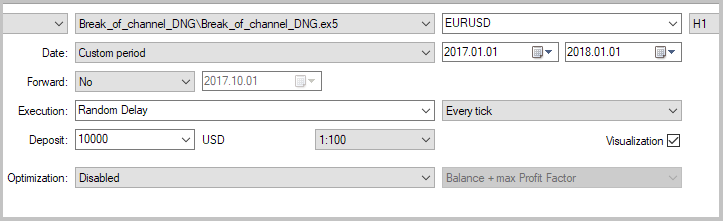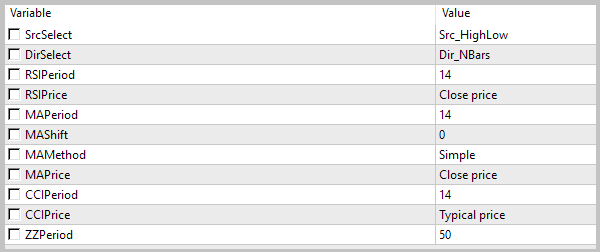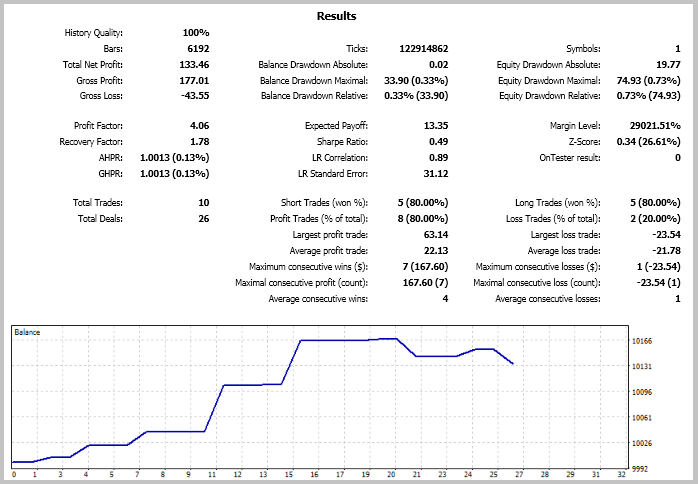#### 4.2. M15 时间帧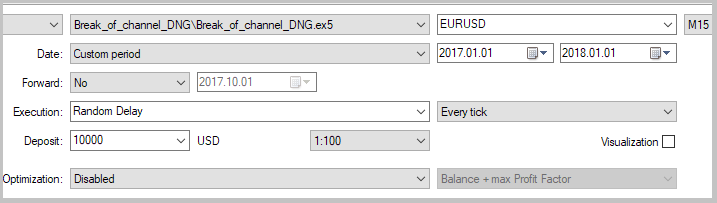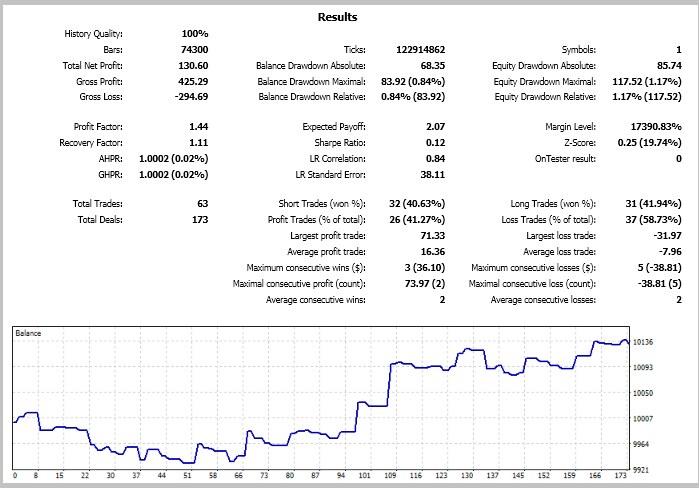#### 4.3. 在其它品种上测试

EURUSD1026804.061.78552
GBPUSD28501.470.232072
EURGBP51400.0-0.71976
USDJPY617830.72-0.19875

EURGBP 货币对得到的结果最糟糕。5 笔交易当中没有一笔盈利平仓。但如果我们分析价格图表, 我们可以看到入场后的潜在盈亏与策略相一致。从以下屏幕截图可以看出, 通道突破策略会生成很好的入场信号。但它需要一个相应的离场策略才能实现更稳定的操作。这可通过持仓时间来确认。测试显示, 平均持仓时间为 550 至 2100 小时, 具体还要取决于品种。市场趋势在如此长的时期内可能会发生多次变化。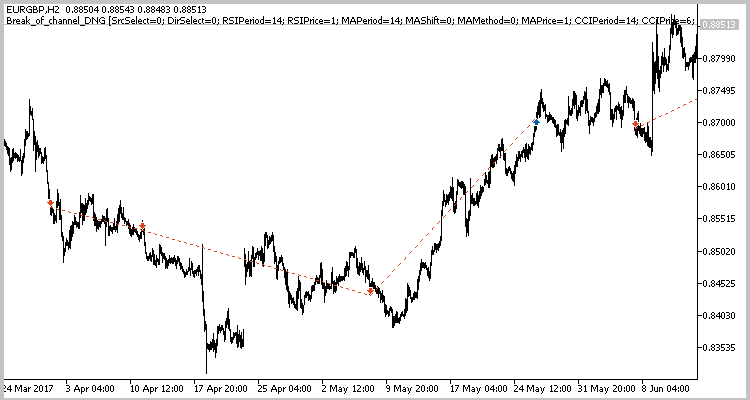### 本文中使用的程序:

#
名称

1Break_of_channel_DNG.mq5 智能交易系统 用于测试策略的智能交易系统
2Channel.mqh 类库 搜索价格通道和开仓信号的类
3Break_of_channel_DNG.mqproj  项目描述文件
4iUniZigZagSW.ex5 指标 通用之字折线
5Reports.zipZip 存档 智能交易系统测试报告

MQL5.zip (204.37 KB)
Reports.zip (241.95 KB)

#### 该作者的其他文章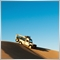| 22 2月 2018 在 03:43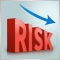如何降低交易者的风险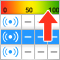自动选择有 "钱途" 的信号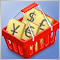测试交易货币对篮子时出现的形态。第三部分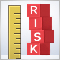单一资产交易顺序中的风险评估. 续篇